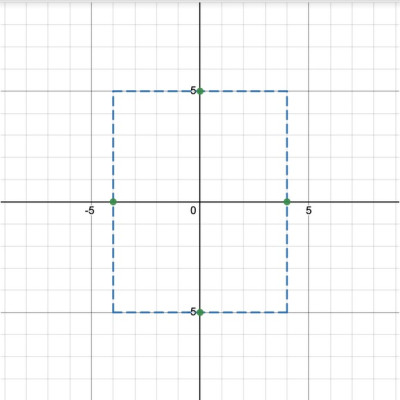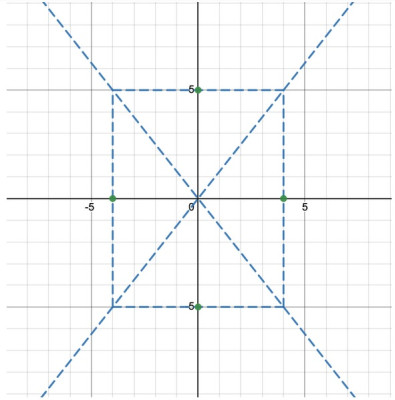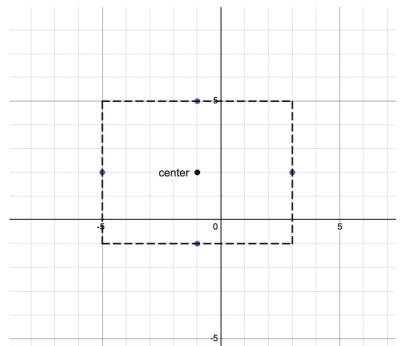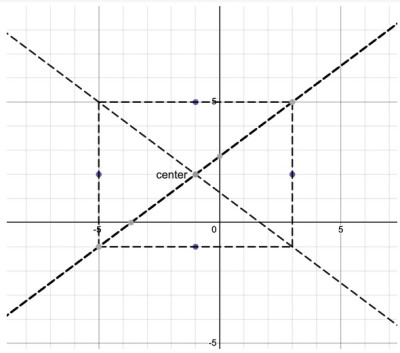# Hyperbolas (3 Key Concepts & Examples)

A hyperbola, in analytic geometry, is a conic section that is formed when a plane intersects a double right circular cone at an angle so that both halves of the cone are intersected. The intersection of the plane and the cone produces two separate unbounded curves that are mirror images of each other called a hyperbola. (Source: cuemath.com)

In this article, we’ll answer common questions about hyperbolas and we’ll look at a few examples to help make the concepts clearer. We’ll also go through the process of graphing hyperbolas.

Having math trouble?

Looking for a tutor?

## What is the equation of a hyperbola?

The equation of a hyperbola describes the set of points where the difference of the distances between 2 fixed points called the foci is constant. That constant is equal to 2a, which is the length of the transverse axis

Note: For hyperbolas, it is very important to recognize if the first term involves x or y. In our equation, x2/a2 is the first term so we have a horizontal transverse axis.

## How do we graph hyperbolas?

### Example

Graph the hyperbola:

(x2/16) – (y2/25) = 1

Solution

In this example, we can see that the denominators are both perfect squares so we can solve for our values of a and b easily enough:

• a2=16 so a=4
• b2=25 so b=5

Since our values of a and b represent lengths, we don’t need to be concerned with the negative square roots. To find the foci, we just need to solve the equation:

• c2 = a2 + b2
• c2 = 42 + 52
• c2 = 16 + 25
• c2 = 41
• c = √41
• c ~ 6.4

So, this means our foci are (6.4, 0) and (-6.4, 0). We can now write the equation of the slant asymptotes

y =(5/4)x and y = -(5/4)x

Putting this all together, we graph the hyperbola step by step. The center of the hyperbola is (0, 0).

Use the values of a and b to draw a rectangle around the center of the hyperbola. This rectangle will help us graph the hyperbola.

Since a is 4, this means the length of the transverse axis is 2a, or 8. Centering the rectangle around the origin, we obtain the vertices (4, 0) and (-4, 0).

Similarly, use b to obtain points (0, 5) and (0, -5) on the conjugate axis. Use these points to draw a rectangle, as below.If we start by drawing a rectangle using a = 4 and b = 5, it will help us to draw the hyperbola itself.

Now, sketch the slant asymptotes. Each asymptote will go through a pair of opposite vertices of our rectangle so we get:Connecting opposite corners of the rectangle and extending the lines gives us the two slant asymptotes.

Putting in the foci, we obtain:

Finally, we’re ready to sketch the hyperbola! Since this is a hyperbola with a horizontal transverse axis, the graph will open left/right. The hyperbola will have symmetry over the y-axis. Use the slant asymptotes and the vertices as graphing aids.

And there we have it!!!

Let’s consider a hyperbola centered at the origin with a vertical transverse axis. As you may have guessed, the equation is similar to the previous one,  but now the equation will start with the y-term.

We can use a similar process as before but now we need to be thinking vertically, instead of horizontally.

For example, here is the graph of the equation (y2/9) – (x2/4) = 1.

Graphing hyperbolas that are centered at the origin are relatively easy. Let’s look at the more general form where we have a center that has been translated.

### Example

Sketch the graph of the hyperbola. Find the center, the slant asymptotes, and the foci.

((y-2)2/9) – ((x+1)2/16) = 1

Solution

First, it’s important to recognize that we have a hyperbola with a vertical transverse axis because the first term in the equation involves y2.

The center of the hyperbola is (h, k). Be careful here. The value of h is always found by looking at the x2 term, which in this case, is the second term.

A common mistake is to assume that the value of h derives from the first term.  Also, the h and k values are the opposite signs of the constants in parentheses.

So, our center is (-1, 2). Thinking in terms of translations, this is a hyperbola that has been translated 1 unit to the left of the origin and 2 units up.

To find the foci, we first need to find the values of a and b. Remember, for the hyperbola equations, the value of a is the square root of the denominator of the first term in the equation. So, here we have a=a = √9=3 and b=√16=4. Now, solve for c.

• c2 = a2 + b2
• c2 = 32 + 42
• c2 = 9 + 16
• c2 = 25
• c = 5

Because we have a hyperbola with a vertical transverse axis, the foci are given by (h, k + c) and (h, kc). In this case, h = -1 and k = 2, so the foci are (-1, 2 + 5) and (-1, 2 – 5), which simplifies to (-1, 7) and (-1, -3).

To help us graph, we’ll use our values a=3 and b=4 to help determine the sketching rectangle. We’ll start by graphing the center of the hyperbola (-1, 2).

We can then add and subtract our value of a to the y-coordinate of the center to find the top and bottom of the transverse axis. This gives us the points (-1, 5) and (-1, -1).

Using b=4, we can add and subtract these values to the x-coordinate of the center to find the points (3, 2) and (-5, 2). Graph these 5 points and draw the rectangle.We begin by drawing a rectangle (using a, b, and the center) to help us draw the hyperbola.

Let’s find the equations of the slant asymptotes and we’ll be ready to graph. In practice, we don’t necessarily need the equations of the asymptotes because they are the lines that pass through opposite vertices of our rectangle which we can simply sketch.

In any case, the equations are found using the equation y=ab(x-h)+k. We already know the values of a, b, h, and k so our equations are y=34(x+1)-2.Connecting opposite corners of the rectangle and extending the lines gives us the two slant asymptotes.

Great, we’re now in good shape to sketch the graph. We can put the foci into the sketch, then use the asymptotes and the vertices to help draw the hyperbola.

And there we have it!!!

Here is the same sketch with a, b, and c labeled.

It’s worth talking about the difference between the equation of a hyperbola versus the equation of another conic section, the ellipse

## How can I tell if the equation is a hyperbola or an ellipse?

Sometimes students get confused when determining whether a conic equation is an ellipse or a hyperbola.

Admittedly, the equations look very similar with one difference: an ellipse is a sum and a hyperbola is a difference. That’s the only difference, but what a difference it makes! Consider the examples below.

## Can the graph of a hyperbola be a function?

No! A function must pass the vertical line test. In the case of a hyperbola with a vertical transverse axis and in the case of a hyperbola with a horizontal transverse axis, the relations will fail the vertical line test! Confirm this yourself by glancing at our example graphs.

## Does a hyperbola have eccentricity?

Yes! Generally speaking, the eccentricity of a conic section measures how curved the shape is. The eccentricity of a hyperbola is given by the formula:

e = √(1 + (b2/a2))

The eccentricity of a hyperbola is always greater than 1.

Now, you should be well versed in graphing hyperbolas!